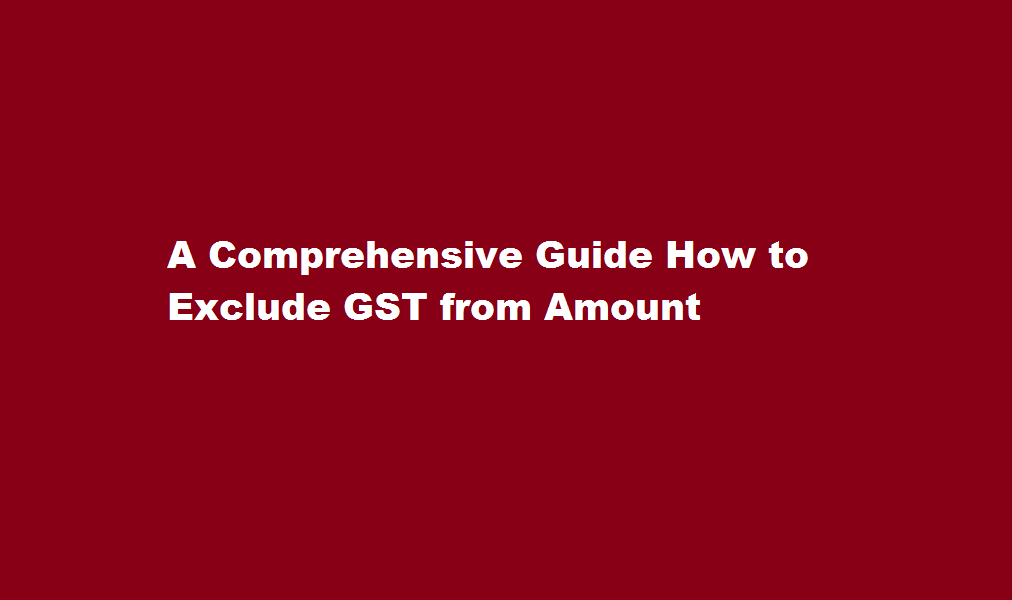December 4, 2023
[stock-market-ticker]

# A Comprehensive Guide How to Exclude GST from Amount## Introduction

Goods and Services Tax (GST) is a consumption tax imposed on the supply of goods and services in many countries. The inclusion of GST in the final amount can sometimes cause confusion, especially when calculating prices or expenses. This article aims to provide a clear and concise guide on how to exclude GST from an amount, ensuring accurate calculations and better financial management.

## Understanding GST

Before delving into the process of excluding GST from an amount, it is crucial to understand the basics of GST. GST is typically applied as a percentage of the total price, added on top of the base value of goods or services. The percentage varies between countries and is usually specified by the government. In most cases, the GST amount is mentioned separately on invoices or receipts.

Also Read  Mastering The Stock Market A Comprehensive Guide to Learning and Succeeding

## Step-by-Step Guide

Identify the GST rate – The first step is to determine the applicable GST rate for the specific goods or services. This rate can vary depending on the country and the nature of the transaction. For instance, some countries may have different GST rates for essential goods, luxury items, and services.

Calculate the GST amount – Once you have identified the applicable GST rate, calculate the GST amount by multiplying the base value of the goods or services by the GST rate (expressed as a decimal). For example, if the base value is \$100 and the GST rate is 10%, the GST amount would be \$10.

Determine the total amount including GST – To calculate the total amount including GST, add the GST amount to the base value. Continuing with the previous example, the total amount including GST would be \$100 + \$10 = \$110.

Reverse calculation – To exclude the GST amount from the total, you need to perform a reverse calculation. Divide the total amount (including GST) by the GST rate plus 1 (expressed as a decimal). In our example, divide \$110 by 1.10 to obtain the base value of \$100.

Also Read  Exploring Poker’s United States Origins

Cross-check the calculation – After performing the reverse calculation, it is essential to cross-check the result. Add the GST amount obtained from step 2 to the base value obtained from step 4. The sum should match the original total amount, ensuring the accuracy of the calculation.

Practical examples – Let’s consider a practical scenario. If you have a bill for \$550 including GST at a rate of 20%, divide \$550 by 1.20 (20% + 1) to obtain the base value of \$458.33. Adding the GST amount of \$91.67 to the base value will yield the original total amount of \$550.

### How do I exclude 7% GST?

When adding 7% to the price is relatively easy (just multiply the amount by 1.07), reverse GST calculations are quite tricky: To work out the price without GST you have to divide the amount by 1.07 (\$214/1.07=\$200)

Also Read  Understanding Taxes When Investing in Foreign Stocks A Comprehensive Guide

### What is the exemption limit for GST?

In India, businesses with an annual turnover of more than Rs. 40 lakhs (Rs. 20 lakhs for businesses in some special category states) are required to register for GST.

## Conclusion

Excluding GST from an amount requires a systematic approach and attention to detail. By following the step-by-step guide provided in this article, you can accurately calculate the base value and exclude GST from the total amount. It is important to understand the applicable GST rate and perform the reverse calculation to ensure the accuracy of the results. By mastering this skill, individuals and businesses can confidently manage their finances, make informed purchasing decisions, and avoid any potential confusion regarding GST-inclusive amounts.